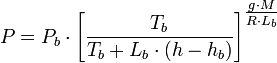# Atmospheric pressure

Atmospheric pressure at any given point in Earth's atmosphere is the downward force per unit area exerted upon a horizontal surface at that point by the weight of air above that surface.

Atmospheric pressure at sea level will vary with geographic location, the temperature and humidity of the air and with the weather conditions. In fact, a change in the sea level atmospheric pressure usually indicates an upcoming change in the weather. Since air temperature and humidity as well as the weather change with the annual seasons (i.e., winter, spring, summer and fall), the sea level atmospheric pressure also changes with the seasons.

##  Standard values of atmospheric pressure at sea level

For more information, see: Reference conditions of gas temperature and pressure and Atmosphere (unit).

In 1954, the 10th Conférence Générale des Poids et Mesures (CGPM) adopted a standard atmosphere for general use and defined it as being precisely 1,013,250 dynes per centimeter (101,325 Pa). This value was intended to represent the average atmospheric pressure at the average sea level at the latitude of Paris, France, and as a practical matter, truly reflects the average sea level pressure for many of the industrialized nations (those with latitudes similar to Paris).

The International standard atmosphere (ISA) as used by International Civil Aviation Organization (ICAO) is also defined as being 101,325 Pa at sea level. The U.S. Standard Atmosphere also defines the sea level atmospheric pressure as being 101,325 Pa.

In chemistry, the original definition of "Standard Temperature and Pressure" by the International Union of Pure and Applied Chemistry (IUPAC) was a reference temperature of 0 °C (273.15 K) and pressure of 101,325 Pa. However, in 1982, the IUPAC recommended that for the purposes of specifying the physical properties of substances, the "standard pressure" should be defined as 100,000 Pa (1 bar).

However, the sea level atmospheric pressure of 101,325 Pa (as defined by the CGPM, the ICAO and also the IUPAC prior to 1982) continues to be very commonly used.

Some equivalent values of the sea level atmospheric pressure (in other units of measurement) are 760 mm-Hg (or 760 torr), 29.92 in-Hg, 1.01325 bar, 14.696 psi and 1013.25 millibars.

As a related matter of interest, the atmosphere (symbol: atm), defined as being 101,325 Pa, is a measurement unit of pressure. The difference between an atm and a bar is about 1%, which is not significant for many applications, and is within the error range of many common pressure gauges.

##  Variation of atmospheric pressure with altitude

Base Values for the U.S. Standard Atmosphere
Region
Number
Altitude Range
(m)
Pb
(Pa)
Tb
(K)
Lb
(K/m)
hb
(m)
1 0.00 to 10,999 101,325 288.15 -0.0065 0.00
2 11,000 to 19,999 22,632 216.65 0.00 11,000
3 20,000 to 31,999 5,474 216.65 0.001 20,000
4 32,000 to 46,999 868 228.65 0.0028 32,000
5 47,000 to 50,999 110 270.65 0.00 47,000
6 51,000 to 70,999 66 270.65 -0.0028 51,000
7 71,000 to 85,000 4 214.65 -0.002 71,000

As the altitude of a point in the atmosphere is increased, the weight of the overlying atmosphere decreases and hence the atmospheric pressure decreases. More simply put, the atmospheric pressure at the top of a tall mountain is lower than the atmospheric pressure at sea level.

The U.S. Standard Atmosphere provides two equations for calculating the atmospheric pressure at any given altitude up to 86 kilometres (53 miles). Equation 1 is used when the lapse rate is not equal to zero and equation 2 is used when the lapse rate equals zero: The two equations are valid for seven different altitude regions of the Earth's atmosphere by using the designated base values (from the adjacent table) for Pb, Tb, Lb and hb for each of the seven regions:

Equation 1:$P = P_b\cdot\left[\frac{T_b}{T_b + L_b\cdot(h - h_b)}\right]^{\textstyle\frac{g\cdot M}{R\cdot L_b}}$
Equation 2:$P = P_b \cdot \exp \left[\frac{-\,g \cdot M \cdot (h-h_b)}{R \cdot T_b}\right]$
 where: P = Atmospheric pressure at any given altitude$h$, Pa Pb = Base atmospheric pressure, Pa Tb = Base atmospheric temperature, K Lb = Base lapse rate, K/m hb = Base height (i.e., altitude), m h = Any given altitude, m g = Gravitational acceleration = 9.8067 m/s2 M = Molecular weight of air = 0.028964 kg/mol R = Universal gas constant = 8.3144 J/(K$\cdot$ mol)

For example, the atmospheric pressure at an altitude of 10,000 metres is obtained as 26,437 Pa by using Equation 1 and the appropriate base values for the altitude region number 1.